Author: Oscar Cronquist Article last updated on June 03, 2019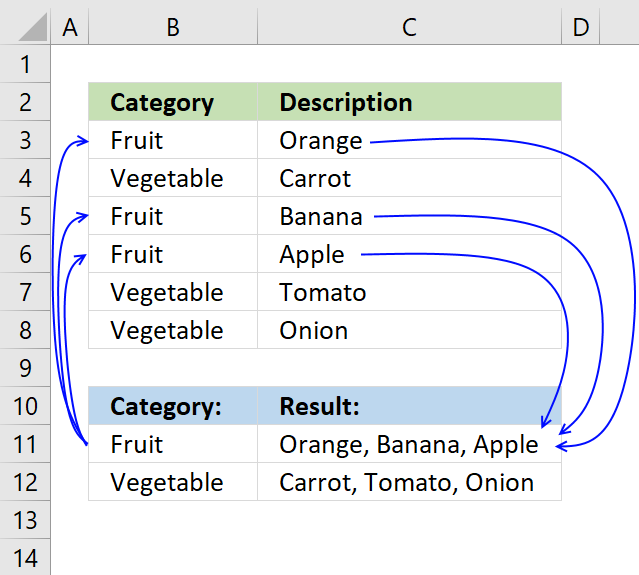This article demonstrates how to find a value in a column and concatenate corresponding values on the same row. The picture above shows an array formula in cell F3 that looks for value "Fruit" in column B and concatenates corresponding values in column C.

The TEXTJOIN function introduced in Excel 365 (subscription required) allows you to easily concatenate values. It also accepts arrays and nested functions.

However if your Excel version is missing the TEXTJOIN function you can use a User Defined Function, I have all instructions on how to do that in this post. The TEXTJOIN function is missing if you don't have the Office 365 subscription service.

Looking for a formula that will take a part number from one column and go and look for all related vehicle applications per that part number and return the vehicle applications to a single cell related back to the part number.

The array formula used in the image above in cell C11 is:

=TEXTJOIN(" ", TRUE, IF(B11=B3:B8, C3:C8, ""))

Here is another example: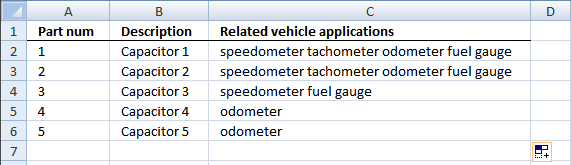Array formula in cell C2:

=TEXTJOIN(" ",TRUE,IF(A2='Vehicle applications'!\$C\$2:\$C\$13,'Vehicle applications'!\$A\$2:\$A\$13,""))

#### Watch a video where I explain the array formula

Recommended article

Concatenate unique distinct values

The new TEXTJOIN function in excel 2016 lets you do some amazing things with arrays. This post demonstrates how to […]

How to enter an array formula

Make sure you enter it as an array formula, follow this:

1. Doubleclick cell C2
2. Paste above formula to cell
3. Press and hold CTRL + SHIFT simultaneously
4. Press enter once
5. Release all keys

If you did this the right way, the formula now has a beginning and ending curly bracket, like this:

{=TEXTJOIN(" ",TRUE,IF(A2='Vehicle applications'!\$C\$2:\$C\$13,'Vehicle applications'!\$A\$2:\$A\$13,""))}

Dont enter these characters yourself, they appear automatically if you follow the instructions above.

Then copy cell C2 and paste to cell range C3:C6.

Recommended article

How to enter an array formula

Array formulas allows you to do advanced calculations not possible with regular formulas.

#### User Defined Function

UPDATE: The solution below is for excel versions that has the TEXTJOIN function missing.

I can´t concatenate values with a formula but I can create a small user defined function.User defined function in cell C2:

=Lookup_concat(A2, 'Vehicle applications'!\$C\$2:\$C\$13, 'Vehicle applications'!\$A\$2:\$A\$13)

+ ENTER

#### Watch a video where I demonstrate the UDF

Picture of sheet "Vehicle applications"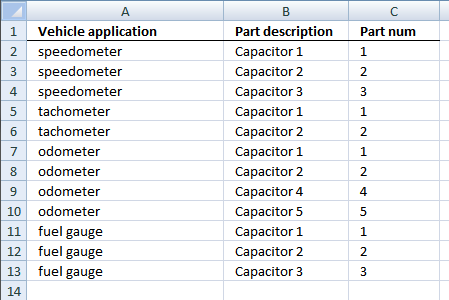#### Explaining user defined function

Lookup_concat(Look_up_value, Search_in_column, Concatenate_values_in_column)
Looks for a value in a column and returns a value on the same row from a column you specify. If multiple values are found the corresponding values are concatenated into a single cell.

See picture below.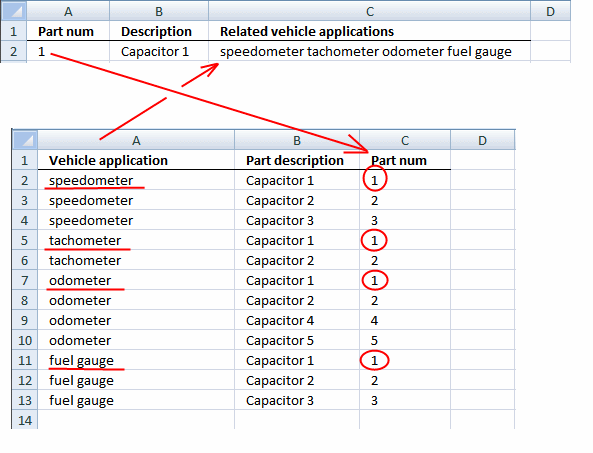#### VBA code

```Function Lookup_concat(Search_string As String, _
Search_in_col As Range, Return_val_col As Range)

Dim i As Long
Dim result As String

For i = 1 To Search_in_col.Count
If Search_in_col.Cells(i, 1) = Search_string Then
result = result &amp; " " &amp; Return_val_col.Cells(i, 1).Value
End If
Next

Lookup_concat = Trim(result)

End Function
```

1. Press Alt-F11 to open visual basic editor
2. Click Module on the Insert menu
3. Copy and paste code above to the code module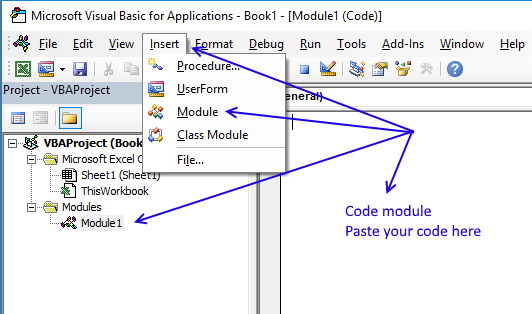4. Exit visual basic editor
5. Save your workbook as a *.xlsm file### Ignore duplicates

The picture below shows you "odometer" found twice in the table but is only displayed once in cell C2. In other words, no duplicates allowed.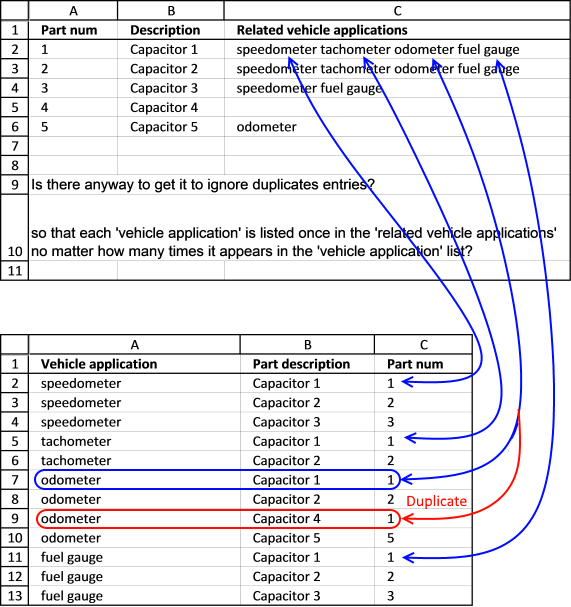Array formula in cell C2:

=TEXTJOIN(", ", TRUE, IF(IFERROR(MATCH('Vehicle applications'!A2:A13, IF(A2='Vehicle applications'!\$C\$2:\$C\$13, 'Vehicle applications'!\$A\$2:\$A\$13, ""), 0),"")=MATCH(ROW('Vehicle applications'!\$A\$2:\$A\$13), ROW('Vehicle applications'!\$A\$2:\$A\$13)), 'Vehicle applications'!\$A\$2:\$A\$13, ""))

Watch a video where I explain the formula

Recommended article

Use a drop down list to filter and concatenate unique distinct values

Question: Is there a way to have a unique list generated from a list? Meaning I have a sheet that […]

How to enter an array formula

#### Explaining array formula in cell C2

Step 1 - Look for a value in a column and return corresponding values

IF(A2='Vehicle applications'!\$C\$2:\$C\$13, 'Vehicle applications'!\$A\$2:\$A\$13, "")

becomes

=IF(1={1; 2; 3; 1; 2; 1; 2; 1; 5; 1; 2; 3}, {"speedometer"; "speedometer"; "speedometer"; "tachometer"; "tachometer"; "odometer"; "odometer"; "odometer"; "odometer"; "fuel gauge"; "fuel gauge"; "fuel gauge"}, "")

and returns

{"speedometer";"";"";"tachometer";"";"odometer";"";"odometer";"";"fuel gauge";"";""}

Step 2 - Match cell range 'Vehicle applications'!A2:A13 with array from step 1

The array in step 1 has a duplicate value, to remove those we need to match the values to the list to find out which values are duplicates.

MATCH('Vehicle applications'!A2:A13, IF(A2='Vehicle applications'!\$C\$2:\$C\$13, 'Vehicle applications'!\$A\$2:\$A\$13, ""), 0)

becomes

MATCH({"speedometer"; "speedometer"; "speedometer"; "tachometer"; "tachometer"; "odometer"; "odometer"; "odometer"; "odometer"; "fuel gauge"; "fuel gauge"; "fuel gauge"}, {"speedometer"; ""; ""; "tachometer"; ""; "odometer"; ""; "odometer"; ""; "fuel gauge"; ""; ""}, 0)

and returns

{1;1;1;4;4;6;6;6;6;10;10;10}

Step 3 - Check if array equals corresponding row number

It is certain that the value is a duplicate if they don't match.

IF(MATCH('Vehicle applications'!A2:A13, IF(A2='Vehicle applications'!\$C\$2:\$C\$13, 'Vehicle applications'!\$A\$2:\$A\$13, ""), 0)=MATCH(ROW('Vehicle applications'!\$A\$2:\$A\$13), ROW('Vehicle applications'!\$A\$2:\$A\$13)), 'Vehicle applications'!\$A\$2:\$A\$13, "")

becomes

IF({1;1;1;4;4;6;6;6;6;10;10;10}=MATCH(ROW('Vehicle applications'!\$A\$2:\$A\$13), ROW('Vehicle applications'!\$A\$2:\$A\$13)), 'Vehicle applications'!\$A\$2:\$A\$13, "")

becomes

IF({1;1;1;4;4;6;6;6;6;10;10;10}= {1;2;3;4;5;6;7;8;9;10;11;12}, 'Vehicle applications'!\$A\$2:\$A\$13, "")

becomes

IF({1; 1; 1; 4; 4; 6; 6; 6; 6; 10; 10; 10}= {1; 2; 3; 4; 5; 6; 7; 8; 9; 10; 11; 12}, {"speedometer"; "speedometer"; "speedometer"; "tachometer"; "tachometer"; "odometer"; "odometer"; "odometer"; "odometer"; "fuel gauge"; "fuel gauge"; "fuel gauge"}, "")

and returns

{"speedometer";"";"";"tachometer";"";"odometer";"";"";"";"fuel gauge";"";""}

Step 4 - Concatenate array

TEXTJOIN(", ", TRUE, IF(MATCH('Vehicle applications'!A2:A13, IF(A2='Vehicle applications'!\$C\$2:\$C\$13, 'Vehicle applications'!\$A\$2:\$A\$13, ""), 0)=MATCH(ROW('Vehicle applications'!\$A\$2:\$A\$13), ROW('Vehicle applications'!\$A\$2:\$A\$13)), 'Vehicle applications'!\$A\$2:\$A\$13, ""))

becomes

=TEXTJOIN(", ", TRUE, {"speedometer";"";"";"tachometer";"";"odometer";"";"";"";"fuel gauge";"";""})

and returns

speedometer, tachometer, odometer, fuel gauge in cell C2.

#### User Defined Functions

If your excel version is missing the TEXTJOIN function you need to add two User Defined Functions to your workbook.

They let you return unique distinct values concatenated into one cell, one UDF creates a unique distinct list and the other UDF concatenates the values.

Watch a video where I explain how to use the UDF:

#### VBA code

```Function Lookup_concat(Search_string As String, _
Search_in_col As Range, Return_val_col As Range)

Dim i As Long
Dim temp() As Variant
Dim result As String
ReDim temp(0)

For i = 1 To Search_in_col.Count
If Search_in_col.Cells(i, 1) = Search_string Then
temp(UBound(temp)) = Return_val_col.Cells(i, 1).Value
ReDim Preserve temp(UBound(temp) + 1)
End If
Next

If temp(0) &lt;&gt;&nbsp;"" Then
ReDim Preserve temp(UBound(temp) - 1)
Unique temp
For i = LBound(temp) To UBound(temp)
result = result &amp; " " &amp; temp(i)
Next i
Lookup_concat = Trim(result)
Else
Lookup_concat = ""
End If

End Function

Function Unique(tempArray As Variant)

Dim coll As New Collection
Dim Value As Variant

On Error Resume Next
For Each Value In tempArray
If Len(Value) &gt;&nbsp;0 Then coll.Add Value, CStr(Value)
Next Value
On Error GoTo 0

ReDim tempArray(0)

For Each Value In coll
tempArray(UBound(tempArray)) = Value
ReDim Preserve tempArray(UBound(tempArray) + 1)
Next Value

End Function

```lookup-and-return-multiple-concatenated-unique-distinct-values.xlsm

Recommended article

How to use the TEXTJOIN function

The TEXTJOIN function is a new function for office 365 subscribers, it is like the CONCATENATE function on steroids or what the CONCATENATE function […]

### Delimiting character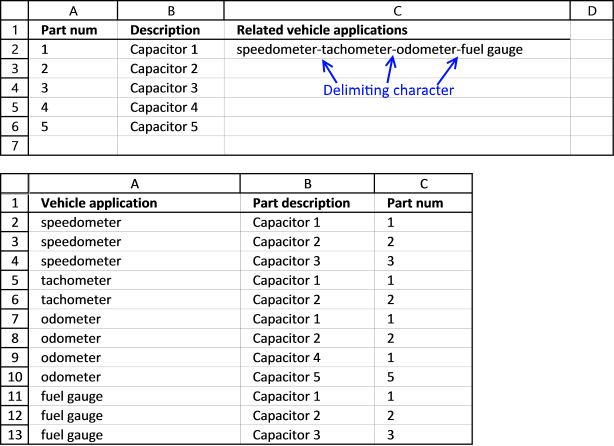The TEXTJOIN function lets you specify a delimiting character in the first argument.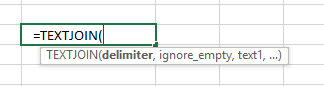This UDF lets you specify a delimiting character in the last argument.

=Lookup_concat(A2,'Vehicle applications'!\$C\$2:\$C\$13,'Vehicle applications'!\$A\$2:\$A\$13,"-")

Watch a video where I explain the UDF

#### VBA Code

```Function Lookup_concat(Search_string As String, _
Search_in_col As Range, Return_val_col As Range, str As String)
Dim i As Long
Dim result As String
For i = 1 To Search_in_col.Count
If Search_in_col.Cells(i, 1) = Search_string Then
result = result &amp; str &amp; Return_val_col.Cells(i, 1).Value
End If
Next
If Len(result) &gt; 0 Then
result = Right(result, Len(result) - Len(str))
Lookup_concat = Trim(result)
Else
Lookup_concat = ""
End If
End Function

```lookup-and-return-multiple-concatenated-values-delimiting-character.xlsm

### Wildcard search

The way this works is that the formula in cell C2 checks if value in cell A2 matches a string in cell range C2:C13. In other words, the whole cell must not match but a sub-string must.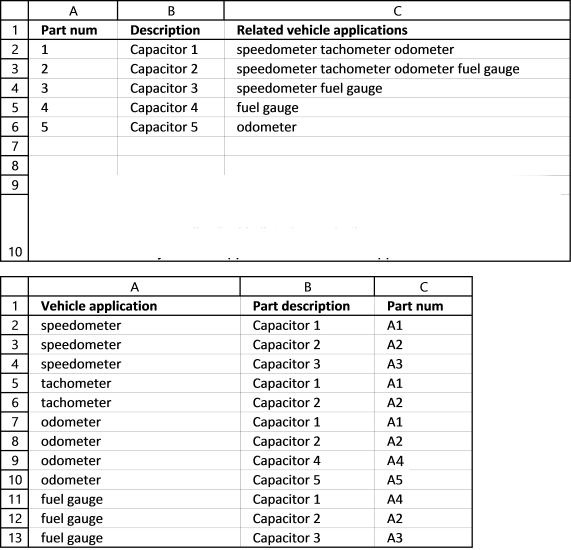Number 1 is found in cell C2,C5 and C7.

Array formula in cell C2:

=TEXTJOIN(",",TRUE,IFERROR(IF(SEARCH(A2,'Vehicle applications'!\$C\$2:\$C\$13),'Vehicle applications'!\$A\$2:\$A\$13,""),""))

Watch a video where I explain the formula

How to enter an array formula

#### Explaining array formula in cell C2

Step 1 - Search for string in cell range

SEARCH(A2,'Vehicle applications'!\$C\$2:\$C\$13)

becomes

=SEARCH(1,{"A1";"A2";"A3";"A1";"A2";"A1";"A2";"A4";"A5";"A4";"A2";"A3"})

and returns

{2; #VALUE!; #VALUE!; 2; #VALUE!; 2; #VALUE!; #VALUE!; #VALUE!; #VALUE!; #VALUE!; #VALUE!}

Step 2 - Return adjacent value

IF(SEARCH(A2,'Vehicle applications'!\$C\$2:\$C\$13),'Vehicle applications'!\$A\$2:\$A\$13,"")

becomes

IF({2; #VALUE!; #VALUE!; 2; #VALUE!; 2; #VALUE!; #VALUE!; #VALUE!; #VALUE!; #VALUE!; #VALUE!},'Vehicle applications'!\$A\$2:\$A\$13,"")

becomes

IF({2;  #VALUE!;  #VALUE!;  2;  #VALUE!;  2;  #VALUE!;  #VALUE!;  #VALUE!;  #VALUE!;  #VALUE!;  #VALUE!},{"speedometer"; "speedometer"; "speedometer"; "tachometer"; "tachometer"; "odometer"; "odometer"; "odometer"; "odometer"; "fuel gauge"; "fuel gauge"; "fuel gauge"},"")

and returns

{"speedometer"; #VALUE!; #VALUE!; "tachometer"; #VALUE!; "odometer"; #VALUE!; #VALUE!; #VALUE!; #VALUE!; #VALUE!; #VALUE!}

Step 3 - Remove errors

IFERROR(IF(SEARCH(A2,'Vehicle applications'!\$C\$2:\$C\$13),'Vehicle applications'!\$A\$2:\$A\$13,""),"")

becomes

IFERROR({"speedometer"; #VALUE!; #VALUE!; "tachometer"; #VALUE!; "odometer"; #VALUE!; #VALUE!; #VALUE!; #VALUE!; #VALUE!; #VALUE!},"")

and returns

{"speedometer";"";"";"tachometer";"";"odometer";"";"";"";"";"";""}

Step 4 - Concatenate values

TEXTJOIN(",",TRUE,IFERROR(IF(SEARCH(A2,'Vehicle applications'!\$C\$2:\$C\$13),'Vehicle applications'!\$A\$2:\$A\$13,""),""))

becomes

TEXTJOIN(",",TRUE,{"speedometer";"";"";"tachometer";"";"odometer";"";"";"";"";"";""})

and returns "speedometer, tachometer, odometer" in cell C2.

#### User Defined Function

For those of you that have an earlier excel version. Watch this vide where I explain how to use it:

#### VBA Code

```Function Lookup_concat(Search_string As String, _
Search_in_col As Range, Return_val_col As Range)

Dim i As Long
Dim temp() As Variant
Dim result As String
ReDim temp(0)

For i = 1 To Search_in_col.Count
If InStr(UCase(Search_in_col.Cells(i, 1)), UCase(Search_string)) Then
temp(UBound(temp)) = Return_val_col.Cells(i, 1).Value
ReDim Preserve temp(UBound(temp) + 1)
End If
Next

If temp(0) &lt;&gt; "" Then
ReDim Preserve temp(UBound(temp) - 1)
For i = LBound(temp) To UBound(temp)
result = result &amp; " " &amp; temp(i)
Next i
Lookup_concat = Trim(result)
Else
Lookup_concat = ""
End If

End Function

```excel-vba-return-multiple-unique-values-into-one-cell-wildcard-searchv2.xlsm

### Searching for the first characters in a text string

The formula and UDF demonstrated below looks for a string that begins with the search string. If search string is a it will match ab, ac but not ba. ab and ac begins with a.

If search string is ab it will match abc, abz but not bca.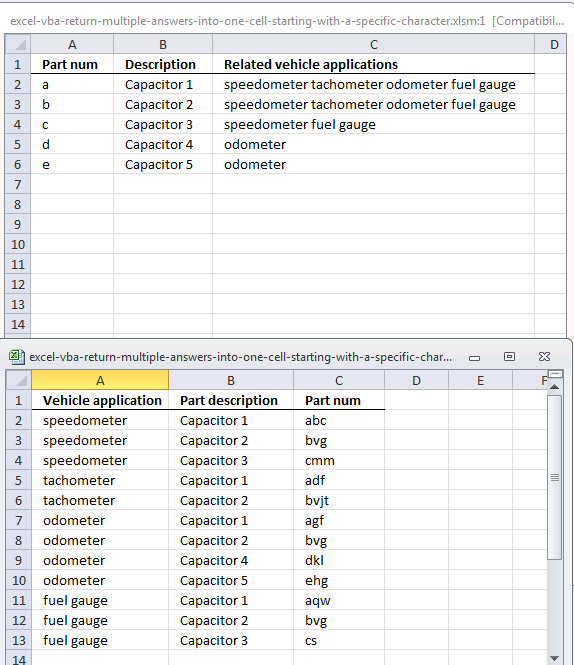Array formula in cell C2:

=TEXTJOIN(",", TRUE, IF(A2=LEFT('Vehicle applications'!\$C\$2:\$C\$13, LEN(A2)), 'Vehicle applications'!\$A\$2:\$A\$13, ""))

Watch a video where I explain the formula

How to enter an array formula

#### Explaining array formula in cell C2

Step 1 - Crop strings so they match search string length

LEFT('Vehicle applications'!\$C\$2:\$C\$13, LEN(A2)

becomes

and returns

{"a";"b";"c";"a";"b";"a";"b";"d";"e";"a";"b";"c"}

Step 2 - Check if "a" is equal to values in array

IF(A2=LEFT('Vehicle applications'!\$C\$2:\$C\$13,LEN(A2)),'Vehicle applications'!\$A\$2:\$A\$13,"")

becomes

IF("a"={"a";"b";"c";"a";"b";"a";"b";"d";"e";"a";"b";"c"},'Vehicle applications'!\$A\$2:\$A\$13,"")

and returns

{"speedometer";"";"";"tachometer";"";"odometer";"";"";"";"fuel gauge";"";""}

Step 3 - Concatenate values

TEXTJOIN(",", TRUE, IF(A2=LEFT('Vehicle applications'!\$C\$2:\$C\$13, LEN(A2)), 'Vehicle applications'!\$A\$2:\$A\$13, ""))

becomes

TEXTJOIN(",", TRUE, {"speedometer";"";"";"tachometer";"";"odometer";"";"";"";"fuel gauge";"";""})

and returns

"speedometer,tachometer,odometer, fuel gauge" in cell C2.

#### User defined function

If your excel version is missing TEXTJOIN function you can easily use the UDF below.

```Function Lookup_concat(Search_string As String, _
Search_in_col As Range, Return_val_col As Range)

Dim i As Long
Dim result As String

For i = 1 To Search_in_col.Count
If Left(Search_in_col.Cells(i, 1), Len(Search_string)) = Search_string Then
result = result &amp; " " &amp; Return_val_col.Cells(i, 1).Value
End If
Next

Lookup_concat = Trim(result)

End Function

```### Lookup and return multiple dates concatenated into one cell

Excel sees dates as numbers so they can be used in formulas and calculations, Jan 1, 1900 is 1 and June 7, 2017 is 42893. To concatenate dates and not numbers we need to use the TEXT function to convert numbers to dates.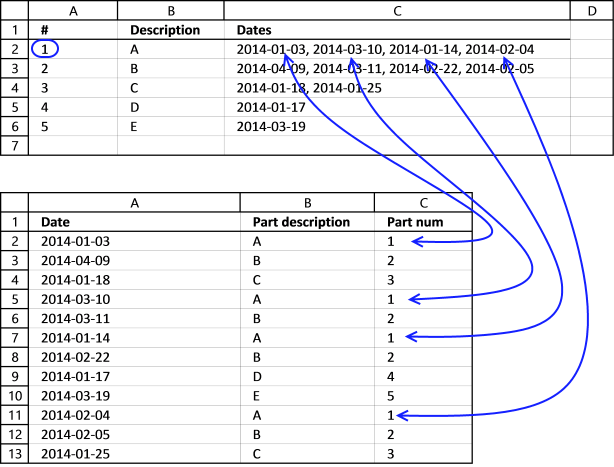(Pic shows dates formatted in this order: YYYY-MM-DD)

Array formula in cell C2:

=TEXTJOIN(", ",TRUE,IF(A2='Vehicle applications'!\$C\$2:\$C\$13,TEXT('Vehicle applications'!\$A\$2:\$A\$13,"YYYY-MM-DD"),""))

Watch a video where I explain the formula

#### Explaining formula in cell C2

Step 1 - Convert datenumbers to dates

TEXT('Vehicle applications'!\$A\$2:\$A\$13,"YYYY-MM-DD")

becomes

TEXT({41642; 41738; 41657; 41708; 41709; 41653; 41692; 41656; 41717; 41674; 41675; 41664},"YYYY-MM-DD")

and returns

{"2014-01-03"; "2014-04-09"; "2014-01-18"; "2014-03-10"; "2014-03-11"; "2014-01-14"; "2014-02-22"; "2014-01-17"; "2014-03-19"; "2014-02-04"; "2014-02-05"; "2014-01-25"}

Step 2 - Check if "a" is equal to values in array

IF(A2='Vehicle applications'!\$C\$2:\$C\$13,TEXT('Vehicle applications'!\$A\$2:\$A\$13,"YYYY-MM-DD"),"")

becomes

IF(A2='Vehicle applications'!\$C\$2:\$C\$13,{"2014-01-03"; "2014-04-09"; "2014-01-18"; "2014-03-10"; "2014-03-11"; "2014-01-14"; "2014-02-22"; "2014-01-17"; "2014-03-19"; "2014-02-04"; "2014-02-05"; "2014-01-25"},"")

becomes

=IF({TRUE;FALSE;FALSE;TRUE;FALSE;TRUE;FALSE;FALSE;FALSE;TRUE;FALSE;FALSE},{"2014-01-03"; "2014-04-09"; "2014-01-18"; "2014-03-10"; "2014-03-11"; "2014-01-14"; "2014-02-22"; "2014-01-17"; "2014-03-19"; "2014-02-04"; "2014-02-05"; "2014-01-25"},"")

and returns

{"2014-01-03";"";"";"2014-03-10";"";"2014-01-14";"";"";"";"2014-02-04";"";""}

Step 3 - Concatenate values

TEXTJOIN(", ",TRUE,IF(A2='Vehicle applications'!\$C\$2:\$C\$13,TEXT('Vehicle applications'!\$A\$2:\$A\$13,"YYYY-MM-DD"),""))

becomes

TEXTJOIN(", ",TRUE,{"2014-01-03";"";"";"2014-03-10";"";"2014-01-14";"";"";"";"2014-02-04";"";""})

and returns

"2014-01-03, 2014-03-10, 2014-01-14, 2014-02-04" in cell C2.

#### User defined function

If your excel version is missing TEXTJOIN function you can use this UDF.

```Function Lookup_concat(Search_string As String, _
Search_in_col As Range, Return_val_col As Range)

Dim i As Long
Dim result As String

For i = 1 To Search_in_col.Count
If Search_in_col.Cells(i, 1) = Search_string Then
result = result &amp; ", " &amp; Return_val_col.Cells(i, 1).Value
End If
Next

Lookup_concat = Right(result, Len(result) - 2)

End Function

```excel-vba-return-multiple-dates-into-one-cell.xlsm

### Lookup within a date range and return multiple values concatenated into one cell

The following formula and UDF use a start and end date to filter dates in col C (table2) and return corresponding items in col A.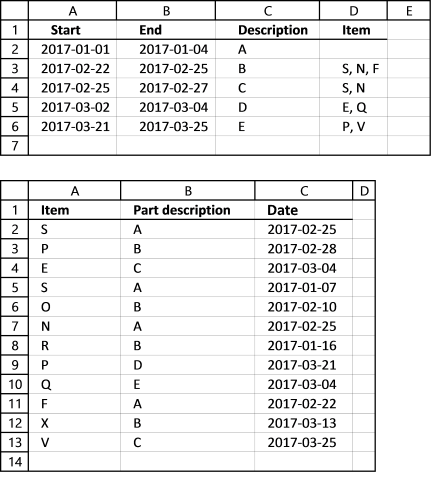Array formula in cell D3 (table1):

=TEXTJOIN(", ", TRUE, IF((A3<='Vehicle applications'!\$C\$2:\$C\$13) *(B3>='Vehicle applications'!\$C\$2:\$C\$13), 'Vehicle applications'!\$A\$2:\$A\$13, ""))

Watch a video where I explain the formula

How to enter an array formula

#### Explaining formula in cell D3

Step 1 - Build logical expression to filter dates in col C (table 2) using start and end dates in table 1

(A3<='Vehicle applications'!\$C\$2:\$C\$13) *(B3>='Vehicle applications'!\$C\$2:\$C\$13)

becomes

(42788<={42791; 42794; 42798; 42742; 42776; 42791; 42751; 42815; 42798; 42788; 42807; 42819}) *(42791>={42791; 42794; 42798; 42742; 42776; 42791; 42751; 42815; 42798; 42788; 42807; 42819})

and returns

{1;0;0;0;0;1;0;0;0;1;0;0}

Step 2 - Return value on same row as matching date

IF((A3<='Vehicle applications'!\$C\$2:\$C\$13) *(B3>='Vehicle applications'!\$C\$2:\$C\$13), 'Vehicle applications'!\$A\$2:\$A\$13, "")

becomes

=IF({1;0;0;0;0;1;0;0;0;1;0;0}, {"S"; "P"; "E"; "S"; "O"; "N"; "R"; "P"; "Q"; "F"; "X"; "V"}, "")

and returns

{"S";"";"";"";"";"N";"";"";"";"F";"";""}

Step 3 - Concatenate values

TEXTJOIN(", ", TRUE, IF((A3<='Vehicle applications'!\$C\$2:\$C\$13) *(B3>='Vehicle applications'!\$C\$2:\$C\$13), 'Vehicle applications'!\$A\$2:\$A\$13, ""))

becomes

TEXTJOIN(", ", TRUE, {"S";"";"";"";"";"N";"";"";"";"F";"";""})

and returns

"S, N, F" in cell D3.

#### User Defined Function

If your excel version is missing TEXTJOIN function you can use this UDF.

```Function Lookup_concat(Search_Start As String, Search_End As String, _
Search_in_col As Range, Return_val_col As Range)

Dim i As Long
Dim result As String

For i = 1 To Search_in_col.Count

If Search_in_col.Cells(i, 1) &lt;= Search_End And Search_in_col.Cells(i, 1) &gt;= Search_Start Then
result = result &amp; Return_val_col.Cells(i, 1).Value &amp; ", "
End If
Next

If Len(result) &gt; 0 Then
Lookup_concat = Left(result, Len(result) - 2)
Else
Lookup_concat = ""
End If

End Function

```lookup-within-a-date-range-and-return-concatenated-values.xlsm

### Split search string using a delimiting character and return multiple matching values concatenated into one cell

This UDF lets you use multiple search strings and fetch corresponding values concatenated to one cell.

Example, search string in cell A2 (table 1) is A+B, the search delimiting character is +. The UDF looks for both A and B in column A in table 2. Cell A2 and A3 matches A and B so the values from column B (Biology and Chemistry) are retrieved and concatenated to cell B2.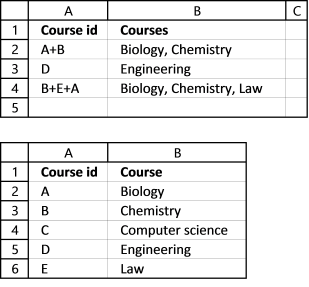Formula in cell B2:

=Lookup_concat(A3,"+",Sheet2!\$A\$2:\$A\$6,", ",Sheet2!\$B\$2:\$B\$6)

This is a User Defined Function so to use the above formula you need to first insert a few lines of code to your workbook.

#### User Defined Function

```Function Lookup_concat(Search_string As String, Search_del As String, _
Search_in_col As Range, Concat_del As String, Return_val_col As Range)
Dim i As Long, j As Long
Dim result As String
Dim srchArr() As String
srchArr = Split(Search_string, Search_del)
For i = 1 To Search_in_col.Count
For j = LBound(srchArr) To UBound(srchArr)
If Search_in_col.Cells(i, 1) = srchArr(j) Then
result = result &amp; Return_val_col.Cells(i, 1).Value &amp; Concat_del
End If
Next j
Next
Lookup_concat = Left(result, Len(result) - Len(Concat_del))
End Function

```

Remember to save your workbook as an *.xlsm file or your code is lost the next time you open your workbook.plit-search-string-using-a-delimiting-character-and-return-multiple-matching-values-concatenated-into-one-cell.xlsm

### Use multiple search values and return multiple matching values concatenated into one cell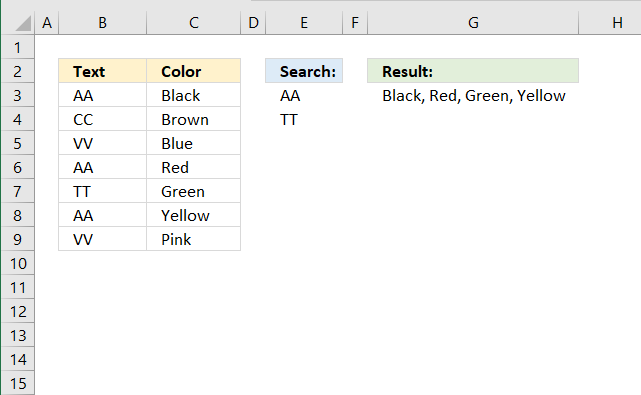Formula in cell G3:

=Lookup_concat(E3:E4,B3:B9,", ", C3:C9)

#### VBA Code

```Function Lookup_concat(Search_string As Range, Search_in_col As Range, Concat_del As String, Return_val_col As Range)
Dim i As Long, j As Long
Dim result As String
For i = 1 To Search_in_col.Count
For Each Value In Search_string
If Search_in_col.Cells(i, 1) = Value Then
result = result & Return_val_col.Cells(i, 1).Value & Concat_del
End If
Next Value
Next i
Lookup_concat = Left(result, Len(result) - Len(Concat_del))
End Function
```